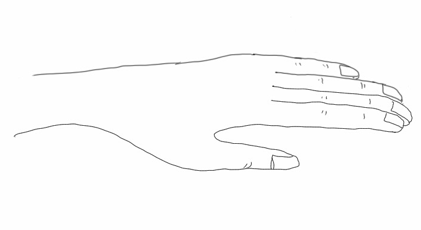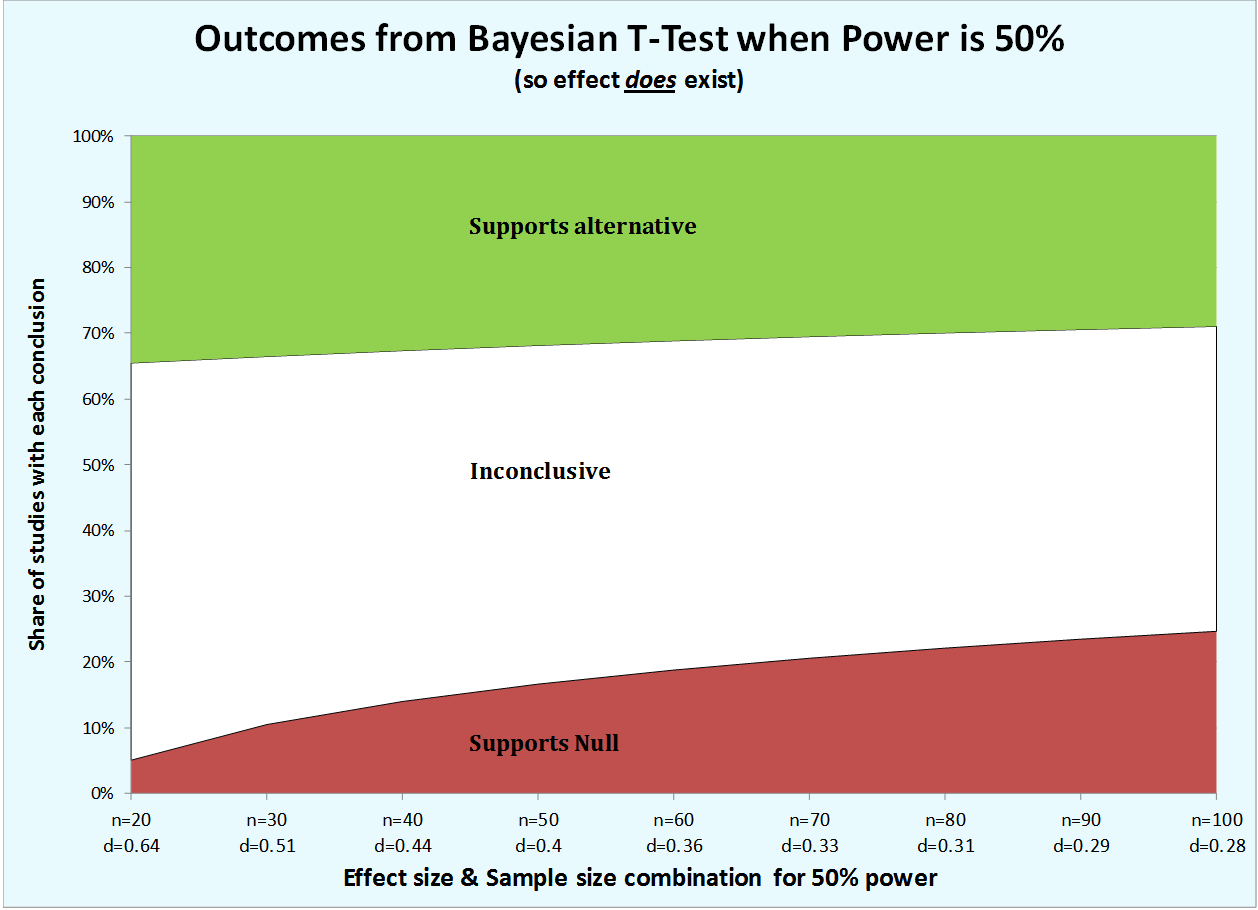# Polish Hand Magic

People often have a hard time remembering their times tables for numbers from 6-9. Polish hand magic (PHM) is a trick for easily calculating the product of any pair of numbers.  Pick two numbers.  Assign one number (l) to your left hand, and the other (r) to your right hand.  Subtract 5 from each number, and hold up that many fingers on the corresponding hand. Then add together the ‘up’ fingers, and multiply by 10.  Add to it the product of the down fingers.

Say you assign 9 to the left hand, and 7 to the right. Hold up 9-5=4 fingers on your left, and 7-5=2 fingers on your right hand.  You have 1 and 3 fingers down, naturally, so:

7*9 is 63.  Neat!  But why on Earth does it work? It’s one of the most frustrating and intriguing examples of hand calculations I’ve ever seen. SMBC expresses how I first felt too, before I came to understand PHM. Nowadays, I still feel PHM is, well, magical–but I also feel like it makes a lot of sense.

# So why does it work?

We can agree that SMBC’s answer is unsatisfying. It’s also representative.  See, for instance, essentially this same explanation here,  here, and here.

But this proof isn’t why it works. We shouldn’t be, and needn’t be, satisfied with the pseudo-answers we get from reflexive algebra.  I’ll show you that there is at least one quite compelling (if surprising) way to think about hand magic, that lets us make algebra that makes sense. I’ll offer essentially the same proof—eventually—but first we’ll imagine the right situation. Once we imagine the situation properly, the algebra—and the whole thing—can be made fairly intuitive.

# Magic relies on redirection

One thing to realize is that like all magic, Polish Hand Magic relies on misdirection.  The real calculations are, in a sense, being hidden from the eye. In order to see around this misdirection  we have to start somewhere totally different. Instead of imagining that we are multiplying numbers, let’s imagine that we are counting fingers—or more precisely, pairs of fingers.  Let’s further imagine that we have 10 fingers on each hand

We want to count how many ways we can pair a finger on our left hand with a finger on our right.  We might pair pinky with pinky, or index finger with thumb, or 8th finger with ring finger.  How many pairs are there?

We can make a table, like this, of all the ways to pair two fingersTable of Finger Pairs: the fingers of the left hand are along the left edge; the fingers of the right hand are along the top, The finger pairs are the little boxes in the middle. (they are labeled based on whether the fingers are up or down. This will be more clear a little later on).

Now, it’s pretty clear from the table that we have 10×10 = 100 pairs of fingers. We can figure it out by counting, or by multiplying. But we can figure this out some other ways too.  I’ll show you that PHM comes from one way to try to count sets like this, using something called the inclusion-exclusion principle.  If we mess this ‘inclusion-exclusion’ method up, we’ll end up calculating l*r.

# Counting Finger Pairs

As an example, let’s say that 6 of the fingers on our left hand are up and 4 are down; our right hand similarly has 8 up and 2 down.  We reason thusly:

“Some of my fingers are up and some are down, so pairs of fingers will have different combinations of up and down fingers.  Some pairs will have only down fingers.  Others will have some up fingers. If I count up each of these types and add them together, I’ll know how many pairs there are.

Now, for a given pair, both fingers might be down.  Since I have 4 down fingers on the left, and 2 on the right, that’s 4×2=8 down pairs.In some pairs, my left finger will be up. Since there are 6 up left fingers, and 10 right fingers all together, that’s 6×10=60 pairs with the left finger up.Similarly, I’ll have 8×10=80 pairs with the right finger up.So that’s

60
80
+8
—–
148 pairs of fingers! Problem solved”.

Of course, you don’t have 148 ways to pair your fingers, you only have 100.

# What went wrong?

There are two things to notice here: (1) The answer we came up with was wrong because we counted some pairs twice.  (2) It was wrong by exactly the product of the up fingers on each hand.

Let’s look again at the key moment of the reasoning.  It was when we counted all pairs with the left finger up, and then  did the same with the right hand. We counted twice the cases where both fingers are up (in the upper left corner of the box).All our counts superimposed on the same table. The upper left section is darker because it was counted twice.

There are exactly 6×8=48 of those, so we got 148.  But hey!  Now we’re on to something.  Say we just counted finger pairs this bad way, and subtracted off the actual number of fingers pairs (100)?  Then we’d have just the overcount—48, which is 6×8.  That’s how PHM works. The only thing left to see is how the 100 gets subtracted off.

# How the hundred got subtracted off.

You don’t really have 10 fingers on each hand.  You have 5.When we do PHM, we don’t multiply 10 by the number of imagined ‘up’ fingers, you do it by the actual number of up fingers, which is five less than the total.  So for each ‘count’, you leave off 5×10 = 50 pairs.  Since you do it for both hands, that means your total count is ‘low’ by 100—assuming you were trying to count the number of finger pairs you could make, if you had 10 fingers on each hand.  But that’s just the product of the original numbers.

# Finally, the proof

Now we’re ready to do our algebra, and understand it.

We start with the number of imaginary up fingers, l and r, that we’d have if we had 10 fingers on each hand, and the number of down fingers, l_d=10-l and r_d = 10-r.

If we did all ten fingers, we’d do

Then we’d have to subtract 100. But instead, we just just the real fingers we have, so we have fewer fingers ‘up’ on each hand: u_l=l-5, and u_r=r-5.

Now we haveAnd that’s it.  And now we know where each piece of the proof came from: the key is trying—and failing—to count the finger pairs of our imaginary hands. The 10 comes because you’re counting finger pairs on your imaginary hands (not because of base 10, which is a total distraction); holding just l-5 and r-5 fingers up is to ‘pre-subtract’ the correct count, 100; the d_r*d_l is to count the pairs that have down fingers.

I find this explanation quite satisfying.  How about you?

Some time soon, I hope to write a little about what I think this explanation says about mathematical understanding in general, and in algebraic derivations in particular.

# When is a test "biased"?

Data Colada recently posted a comment (written by Uri Simonsohn) on a supposed ‘bias’ lurking a common default Bayesian alternative to the t-test.  Point #1 was that the Bayesian T-test is ‘biased’ against low power results. Several smart people have made sophisticated and pretty sensible critiques of Simonsohn’s arguments, which I won’t rehash here. Instead, I want to point out the obvious problem: the claim of ‘bias’ is based on choosing the sample size in a way that is, well, biased.

Here’s the central graph under contention.Now, there are several things to notice. First, not only does the probability that the results support the null increase as the effect size goes down, the probability of the results supporting the alternative go down as well. This is barely noticeable in this graph, but that’s just a function of the particular power level the author chose.  With a power of 0.85 (which is a better estimate of what a person ought to be doing in their experiment anyway), the size of these changes is reversed; the probability of supporting the alternative decreases 5 points from .73 to .68, while the increase in the probability of supporting the null increases just 4 points, from .005 to .042.  I got these numbers using Simonsohn’s publicly available R code).  So it seems that only really low power tests are ‘biased’ against small effect sizes?

But this brings up the deeper question: why make this comparison at all? In particular, why use the notion of ‘power’ to set your sample size?   ‘Power’ is supposed to control for type II errors in a t test.  Why should it have any particular privileged status in setting the size of an experiment?  The answer is that it shouldn’t.  These two concepts have been juxtaposed here without any clear justification.  One could just  as easily choose sample sizes to keep a constant probability that the default test favors the null.  Then one would find that the t-test is ‘biased’ against large effect sizes, in that the power would be higher for small effects.

I might be missing something, but I don’t see what. You have different ways of using models to estimate the parameters for an experiment to control the probabilities of certain kinds of errors.  Parameters that control for one type of error won’t necessarily control for another, but it’s rather extreme to call this a ‘bias’.